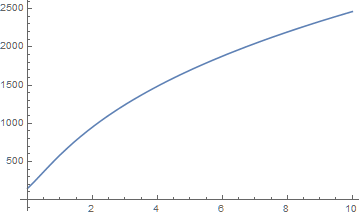# How do I calculate mortgages and loans using annuities?

The TimeValue expression can describe the present value of an Annuity in terms of the cost of each payment, the number of periods, the size of the intervals, and the interest rate.

``` TimeValue[Annuity[payments, periods, intervals], interest, 0] ```

To find the monthly payments on a mortgage with value, equate the present value with some value:

``` TimeValue[Annuity[payments, periods, intervals], interest, 0] == value ```

Use the function Solve to solve the equation in terms of payments:

``` Solve[TimeValue[Annuity[payments, periods, intervals], interest, 0] == value, payments] ```

This returns an algebraic answer for the value of the payments in terms of the other variables. Solve outputs the result as a list of rules.

Solve can calculate with numbers as well. For example, to calculate the payments on a \$200,000 mortgage amortized over 30 years at 5.2% nominal interest, evaluate:

``` Solve[TimeValue[Annuity[payments, 30, 1/12], EffectiveInterest[.052, 1/12], 0] == 200000, payments] ```

```{{payments -> 1098.22}} ```

We can solve for other parameters as well, such as the number of payments. To find the number of years required to pay off a \$10,000 loan with payments of \$200 per month at an 8% interest rate, evaluate:

``` Solve[TimeValue[Annuity[200,periods, 1/12], .08, 0] == 10000,periods] ```

```{{periods -> 5.04369}} ```

To calculate the monthly payments needed to pay off a \$5,000 loan in 3 years, evaluate:

``` Solve[TimeValue[Annuity[payments, 3, 1/12], .08, 0] == 5000, payments] ```

```{{payments -> 156.039}} ```

A loan of \$3,000 is repaid with quarterly installments at the end of each quarter for 5 years. If the interest rate is 10% compounded semiannually, the amount of each quarterly payment is found with the following code:

``` Solve[TimeValue[Annuity[payments, 5, 1/4], EffectiveInterest[.1, 1/2], 0] == 3000, payments] ```

```{{payments -> 191.888}} ```

Solve can also solve for the relationship between two parameters. For example we can find the function relating payments to the interest rate and then plot the relationship:

``` relationship = First[Solve[ TimeValue[Annuity[payments, 5, 1/4], interest, 0] == 3000, payments]]```

``` ```

```Plot[payments /. relationship, {interest, 0, 10}] ```설명이 도움이 되었나요?

하실 말씀이 있습니까?

피드백 감사합니다.

## 지원 문의

청구서, 제품 동기화에 관한 질문에서 기술적인 질문까지 부담없이 문의하세요.전화하기

월요일 - 금요일
8am–5pm 중부 표준시

• 제품 등록 및 동기화
• 구매 전 정보 및 주문
• 설치 및 동작

## 고급 기술지원 (해당 고객을 대상으로)

월요일 - 목요일
8am–7pm 중부 표준시

금요일
8:30–10am & 11am–5pm 중부 표준시

• 우선적 기술지원
• Wolfram 전문가들의 제품 지원
• Wolfram 프로그래밍
• 고급 설치 지원